Question

# Determine the critical​ t-scores for each of the conditions below. ​a) ​one-tail test, alphaαequals=0.01, and nequals=38...

Determine the critical​ t-scores for each of the conditions below.

 ​a) ​one-tail test, alphaαequals=0.01, and nequals=38 ​b) ​one-tail test, alphaαequals=0.005, and nequals=10 ​c) ​two-tail test, alphaαequals=0.05 and nequals=25 ​d) ​two-tail test, alphaα=0.10 and n=13

​a) The critical​ t-score(s) for a​ one-tail test, where

alphaα=0.01​,

and

n=38

​is(are)

nothing.

​(Round to three decimal places as needed. Use a comma to separate answers as​ needed.)

​b) The critical​ t-score(s) for a​ one-tail test, where

alphaα=0.005

and

n=10

​is(are)

nothing.

​(Round to three decimal places as needed. Use a comma to separate answers as​ needed.)

​c) The critical​ t-score(s) for a​ two-tail test, where

alphaα=0.05​,

and

n=2

​is(are)

nothing.

​(Round to three decimal places as needed. Use a comma to separate answers as​ needed.)

​d) The critical​ t-score(s) for a​ two-tail test, where

alphaαequals=0.10

and

n=13

​is(are)

nothing.

​(Round to three decimal places as needed. Use a comma to separate answ

Solution :

Given that,

(a)

sample size = n = 38

Degrees of freedom = df = n - 1 = 38 - 1 = 37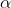= 0.01

t,df = t 0.01,37 = 2.431

critical t score = 2.431

(b)

sample size = n = 10

Degrees of freedom = df = n - 1 = 10 - 1 = 9= 0.005

t,df = t 0.005,9 = 3.250

critical t score = 3.250

(c)

sample size = n = 2

Degrees of freedom = df = n - 1 = 2 - 1 = 1= 0.05/ 2 = 0.05 / 2 = 0.025

t/2 ,df = t 0.025,1 = -/+12.706

critical t score = -12.706 , +12.706

(d)

sample size = n = 13

Degrees of freedom = df = n - 1 = 13 - 1 = 12= 0.10/ 2 = 0.10 / 2 = 0.05

t/2 ,df = t 0.05,12 = -/+1.782

critical t score = -1.782 , +1.782

#### Earn Coins

Coins can be redeemed for fabulous gifts.

Similar Homework Help Questions
• ### Determine the critical t-scores for each of the conditions below. a) one-tail test, α-0.005, and n-39...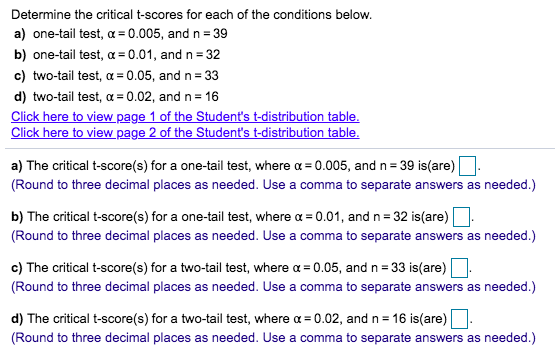Determine the critical t-scores for each of the conditions below. a) one-tail test, α-0.005, and n-39 b) one-tail test, a 0.01, and n 32 c) two-tail test, a 0.05, and n 33 d) two-tail test, a-0.02, and n 16 Click here to view page 1 of the Student's t-distribution table Click here to view page 2 of the Student's t-distribution table a) The critical t-score(s) for a one-tail test, where a-0.005, and n 39 is(are) Round to three decimal places...

• ### Determine the critical t-scores for each of the conditions below. a) one-tail test, a=0.01, and n=18...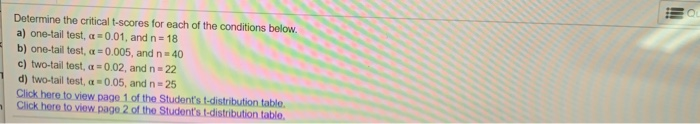Determine the critical t-scores for each of the conditions below. a) one-tail test, a=0.01, and n=18 b) one-tail test, c = 0.005, and n = 40 c) two-tail test, a = 0.02, and n=22 d) two-tail test, a 0.05, and n=25 Click here to view page 1 of the Student's t-distribution table. Click here to view page 2 of the Student's t-distribution table.

• ### For each of the following​ situations, find the critical​ value(s) for z or t. ​a)H0​: rhoρequals=0.30...

For each of the following​ situations, find the critical​ value(s) for z or t. ​a)H0​: rhoρequals=0.30 vs. HA​: rhoρnot equals≠0.30 at alphaαequals=0.01 ​b)H0​: rhoρequals=0.20 vs. HA​: rhoρgreater than>0.20 at alphaαequals=0.10 ​c)H0​: muμequals=30 vs. HA​: muμnot equals≠30 at alphaαequals=0.10 ; n = 47 ​d)H0​: rhoρequals=0.3 vs. HA​: rhoρgreater than>0.3 at alphaαequals=0.05 ; n =350 e)H0​: muμequals=40 vs. HA​: muμless than<40 at alphaαequals=0.10 ​; n=1000 ​a) The critical​ value(s) is(are) z* equals=2.58,−2.58. ​(Use a comma to separate answers as needed. Round to...

• ### Find the critical z values. Assume that the normal distribution applies. alphaαequals=0.030.03​; Upper H 1H1 is...

Find the critical z values. Assume that the normal distribution applies. alphaαequals=0.030.03​; Upper H 1H1 is pless than<0.280.28 zequals=nothing ​(Round to two decimal places as needed. Use a comma to separate answers as​ needed.)

• ### Determine the appropriate critical value(s) for each of the following tests concerning the population mean: a....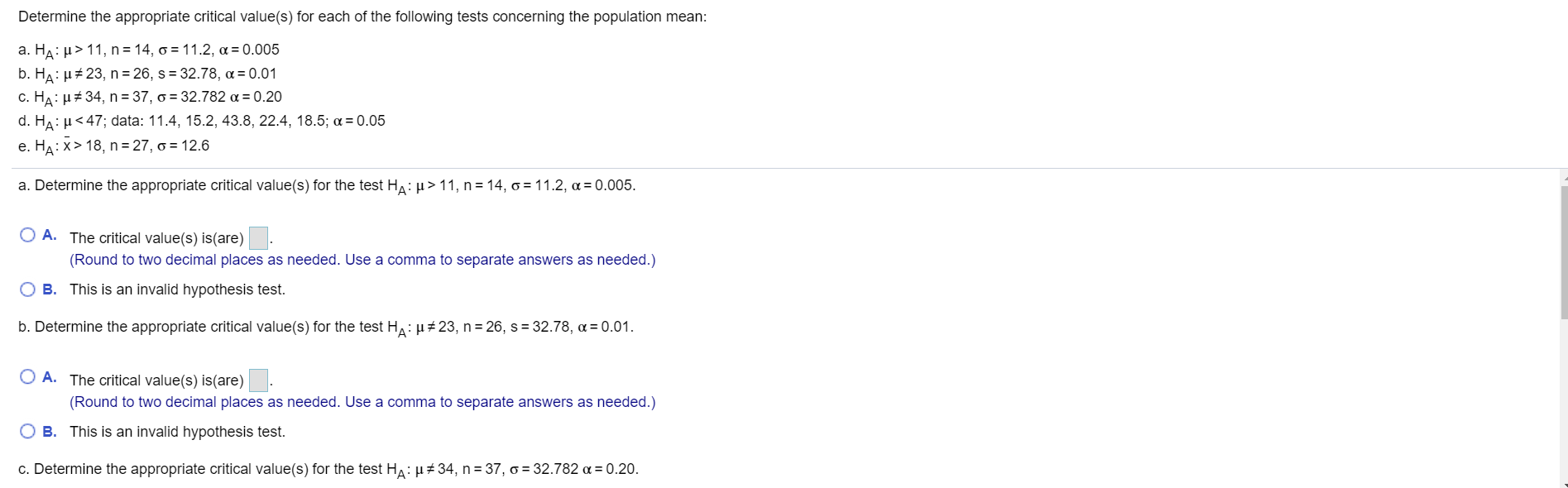Determine the appropriate critical value(s) for each of the following tests concerning the population mean: a. Ha:p> 11, n = 14, o = 11.2, a = 0.005 b. Ha: u # 23, n = 26, s = 32.78, a = 0.01 C. HA: u 34, n = 37, o = 32.782 a = 0.20 d. Ha: < 47; data: 11.4, 15.2, 43.8, 22.4, 18.5; a = 0.05 e. HA:x>18, n=27, o = 12.6 a. Determine the appropriate critical value(s) for...

• ### Complete parts (a) through (c) below. (a) Determine the critical value(s) for a right-tailed test of...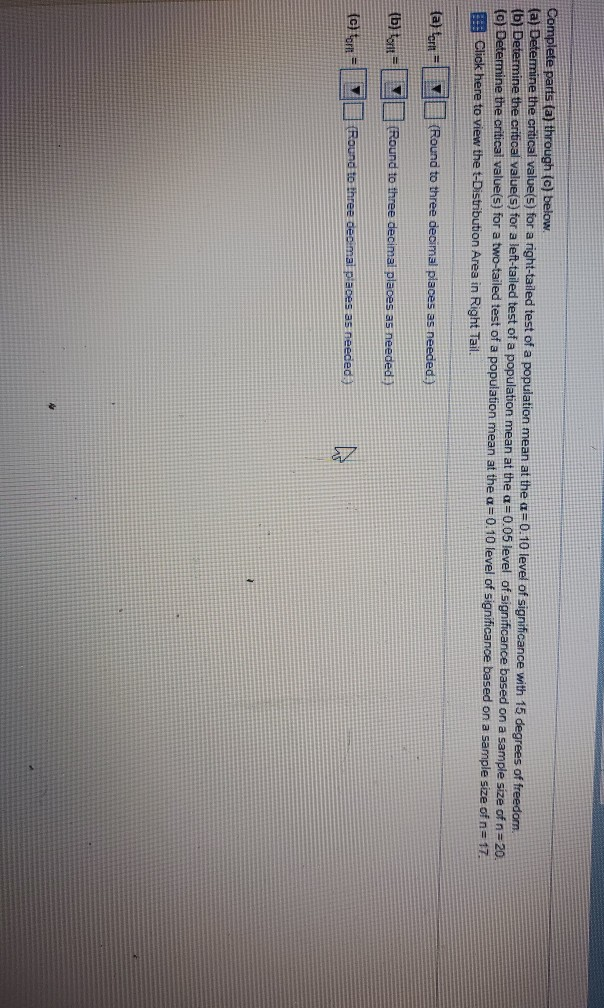Complete parts (a) through (c) below. (a) Determine the critical value(s) for a right-tailed test of a population mean at the a=0.10 level of significance with 15 degrees of freedom. (b) Determine the critical value(s) for a left-tailed test of a population mean at the a=0.05 level of significance based on a sample size of n = 20. (c) Determine the critical value(s) for a two-tailed test of a population mean at the a=0.10 level of significance based on a...

• ### very stuck pls help! For each of the following situations, find the critical value(s) for z...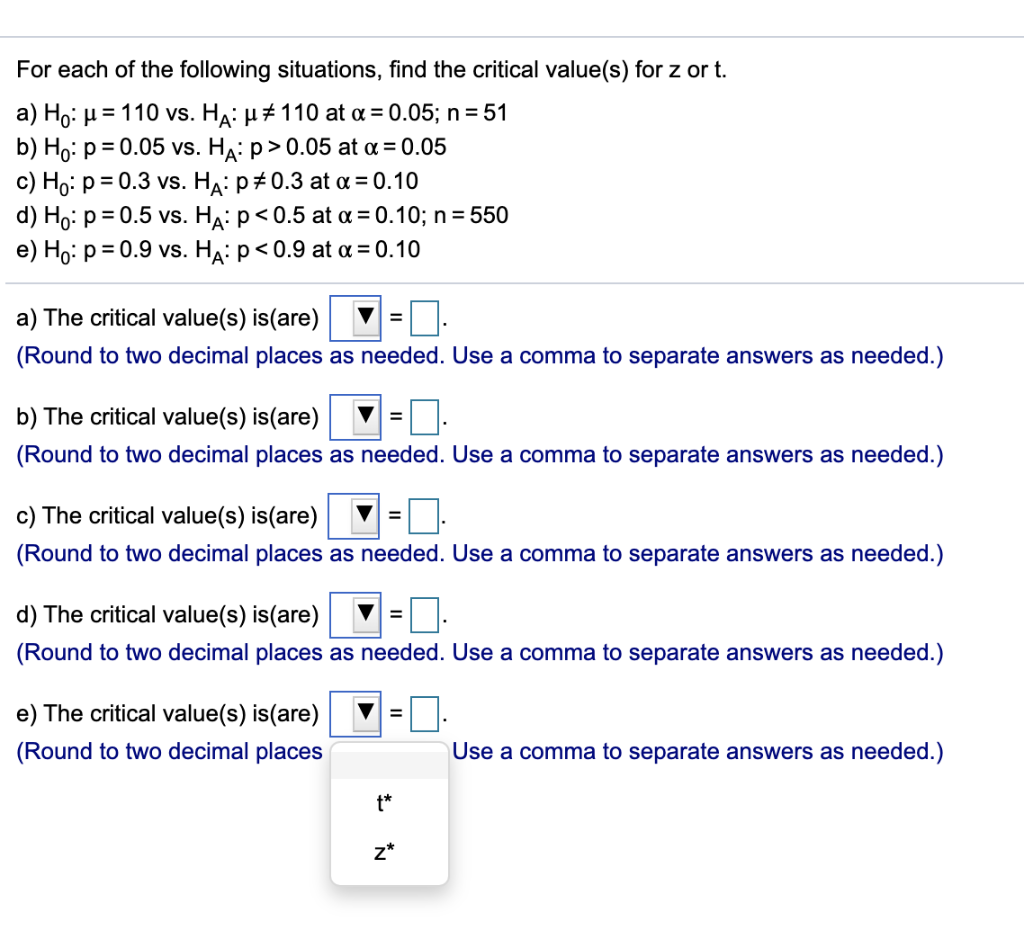very stuck pls help! For each of the following situations, find the critical value(s) for z or t. a) Ho: u = 110 vs. Ha: u# 110 at a = 0.05; n = 51 b) Ho: p = 0.05 vs. Ha:p>0.05 at a = 0.05 c) Ho: p = 0.3 vs. Ha:p*0.3 at a = 0.10 d) Ho: p = 0.5 vs. Ha:p<0.5 at a = 0.10; n = 550 e) Ho: p = 0.9 vs. Ha: p<0.9 at a...

• ### In a one-tail hypothesis test where you reject He only in the lower tail, what is...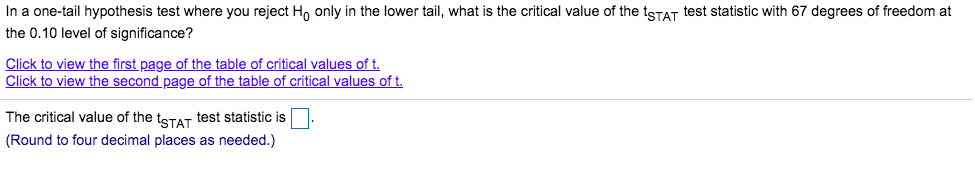In a one-tail hypothesis test where you reject He only in the lower tail, what is the critical value of the Stat test statistic with 67 degrees of freedom at the 0.10 level of significance? Click to view the first page of the table of critical values of t. Click to view the second page of the table of critical values of t. The critical value of the statest statistic is (Round to four decimal places as needed.)

• ### For each of the following situations, find the critical value(s) for z or t. a) Ho:...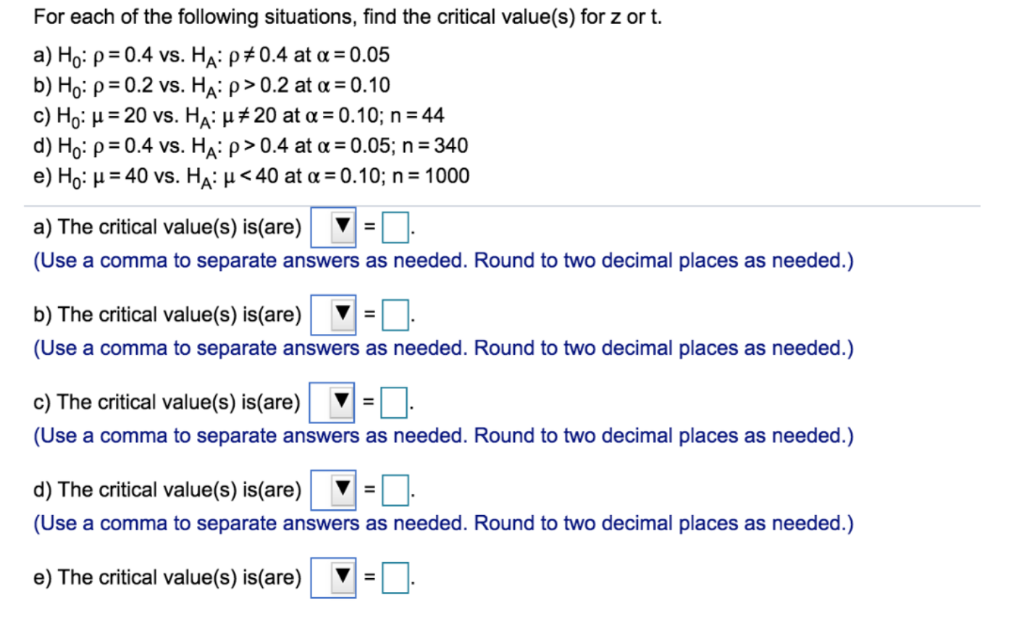For each of the following situations, find the critical value(s) for z or t. a) Ho: ρ:0.4 vs. HA: ρ#0.4 at α-0.05 b) Ho: ρ:0.2 vs. HA: ρ > 0.2 at α-o10 c) Ho: μ-20 vs. HA: μ #20 at α-0.10; n-44 d) Ho: ρ-o4 vs. HA: ρ > 0.4 at α-o05; n-340 e) Ho: μ-40 vs. HA: μ < 40 at α-0.10; n-1000 a)The critical value(s) is(are) ▼ (Use a comma to separate answers as needed. Round to two...